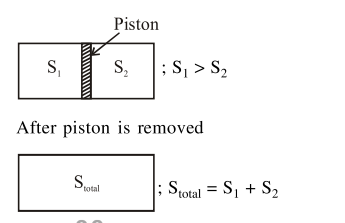# An ideal gas in a cylinder is separated by`
Question:

An ideal gas in a cylinder is separated by a piston in such a way that the entropy of one part is $S_{1}$ and that of the other part is $S_{2}$. Given that $\mathrm{S}_{1}>\mathrm{S}_{2}$. If the piston is removed then the total entropy of the system will be :

1. $S_{1} \times S_{2}$

2. $S_{1}-S_{2}$

3. $\frac{S_{1}}{S_{2}}$

4. $S_{1}+S_{2}$

Correct Option: , 4

Solution: